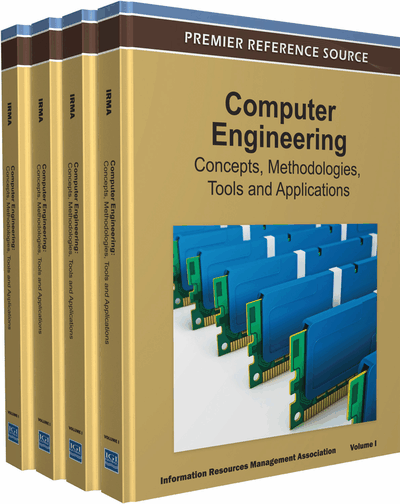# Forward and Backward Chaining with P Systems

Sergiu Ivanov (Academy of Sciences of Moldova and Technical University of Moldova, Moldova), Artiom Alhazov (Academy of Sciences of Moldova, Moldova and Università degli Studi di Milano-Bicocca, Italy), Vladimir Rogojin (Vladimir Rogojin, Helsinki University,  Finland and Academy of Sciences of Moldova, Moldova) and Miguel A. Gutiérrez-Naranjo (Academy of Sciences of Moldova, Moldova and University of Sevilla, Spain)
DOI: 10.4018/978-1-61350-456-7.ch610

## Abstract

One of the concepts that lie at the basis of membrane computing is the multiset rewriting rule. On the other hand, the paradigm of rules is profusely used in computer science for representing and dealing with knowledge. Therefore, establishing a “bridge” between these domains is important, for instance, by designing P systems reproducing the modus ponens-based forward and backward chaining that can be used as tools for reasoning in propositional logic. In this paper, the authors show how powerful and intuitive the formalism of membrane computing is and how it can be used to represent concepts and notions from unrelated areas.
Chapter Preview
Top

## 1. Introduction

The use of rules is one of the most common paradigms in computer science for dealing with knowledge. Given two pieces of knowledge V and W, expressed in some language, the rule V → W is usually considered as a causal relation between V and W. This representation is universal in science. For example, in chemistry, V and W can be metabolites and V → W a chemical reaction. In this case, V represents the reactants which are consumed in the reaction and W is the obtained product. In propositional logic, V → W, with V = v1v2∨…∨ vn and W = w1w2∨…∨ wm, is a representation of the clause –v1 ∨ ­v2 ∨…∨ ­vnw1w2∨…∨ wm.

An important problem is deriving new knowledge: given a knowledge base KB = (A, R), where A is a set of known atoms and R is a set of rules of type V → W, the problem is to know if a new atom g can be obtained from the known atoms and rules. We will call this problem a reasoning problem and it will be denoted by 〈A, R, g〉.

In computer science, there are two basic methods for seeking a solution of a reasoning problem, both of them based on the inference rule known as Generalized Modus Ponens: the former is data-driven and it is known as forward chaining, the latter is query-driven and it is called backward chaining (Apt, 1990).

As one should observe, even though logic inference rules and multiset rewriting rules originate from totally different areas of mathematics and computer science and represent unrelated notions, their concepts have some similarities. In particular, no information about the ordering of elements in both left- and right-hand sides of the rules of both types is used. On the other hand, the inference rules could be thought of as set rewriting rules, while multiset rewriting rules operate at multisets. However, multiset rewriting rules could be interpreted as set rewriting rules if one ignores the multiplicity of elements of the multiset. Therefore we could represent sets of facts in P systems as multisets of objects and inference rules as multiset rewriting rules. When one considers the set of facts represented in a region of a P system, one only considers the underlying set of the region’s multiset.

## Complete Chapter List

Search this Book:
Reset